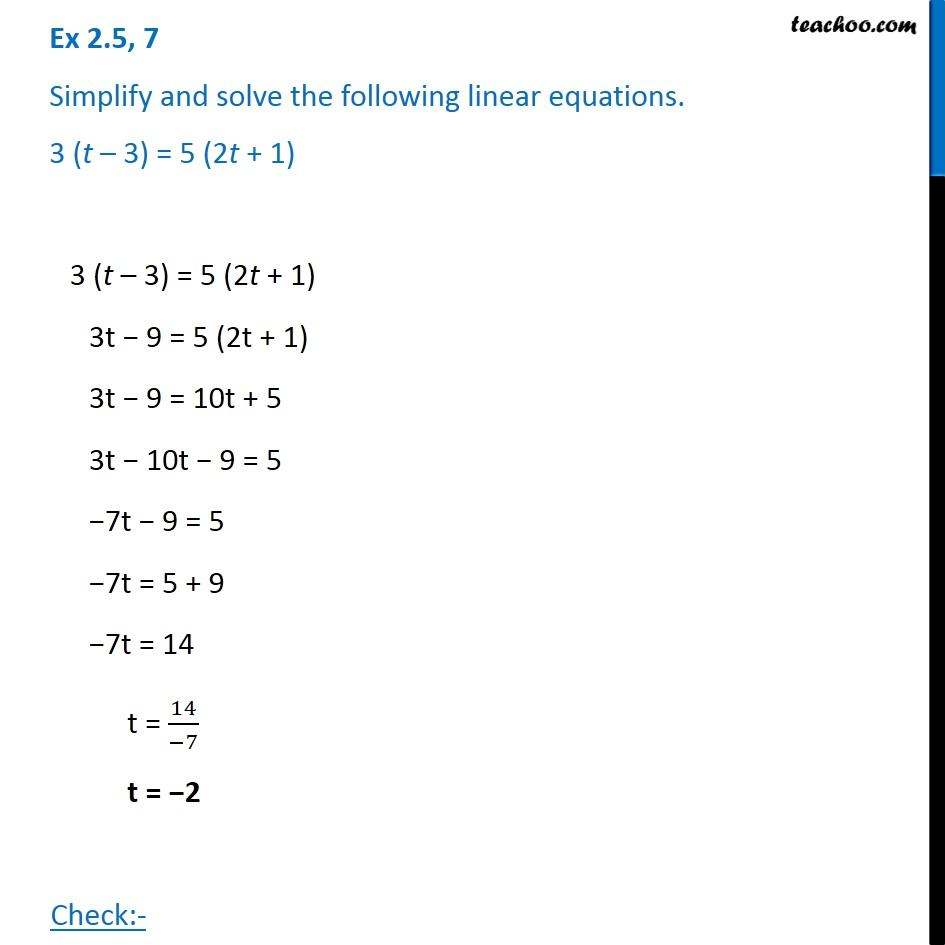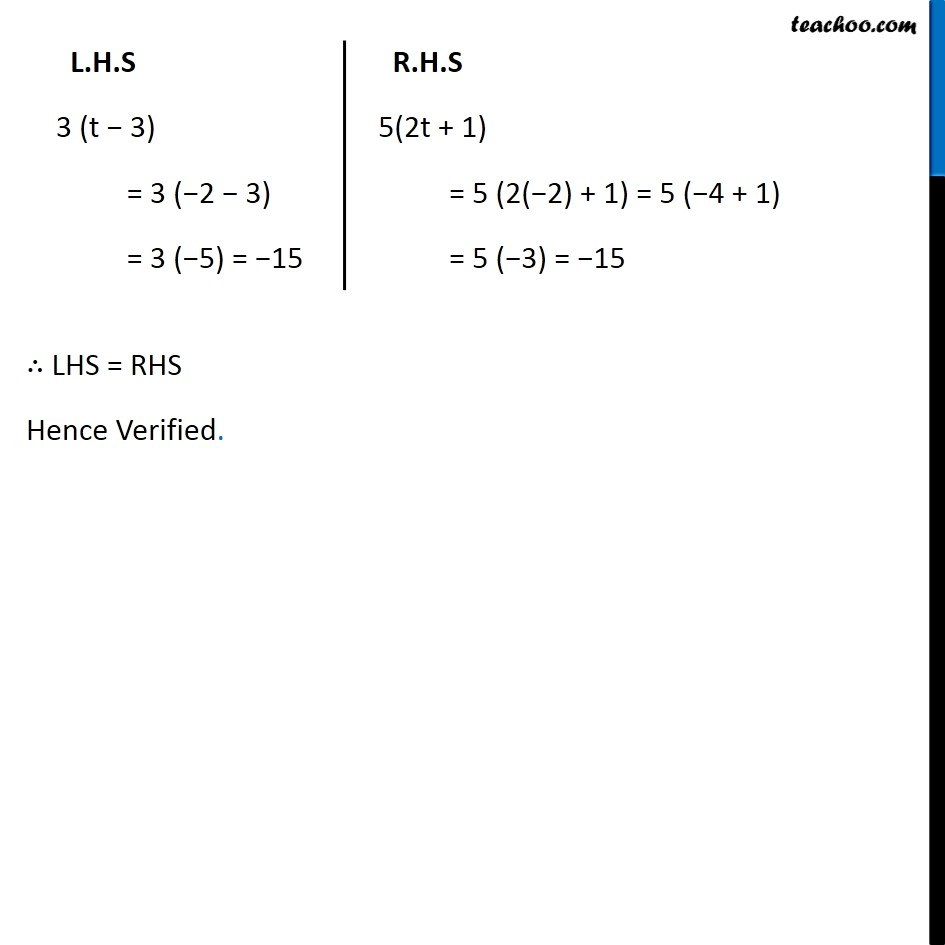1. Chapter 2 Class 8 Linear Equations in One Variable
2. Concept wise
3. Solving Linear Equations - Making equation simpler and then solving

Transcript

Ex 2.5, 7 Simplify and solve the following linear equations. 3 (t – 3) = 5 (2t + 1) 3 (t – 3) = 5 (2t + 1) 3t − 9 = 5 (2t + 1) 3t − 9 = 10t + 5 3t − 10t − 9 = 5 −7t − 9 = 5 −7t = 5 + 9 −7t = 14 t = 14/(−7) t = −2 Check:- L.H.S 3 (t − 3) = 3 (−2 − 3) = 3 (−5) = −15 R.H.S 5(2t + 1) = 5 (2(−2) + 1) = 5 (−4 + 1) = 5 (−3) = −15 ∴ LHS = RHS Hence Verified.